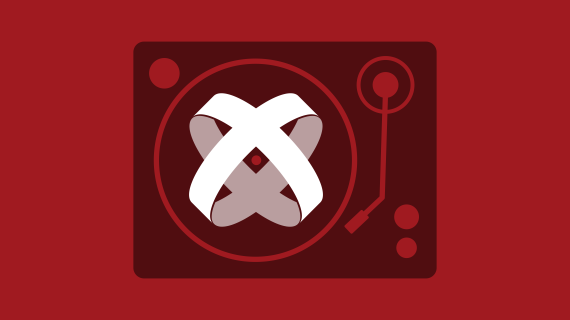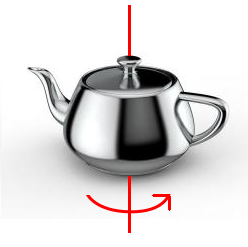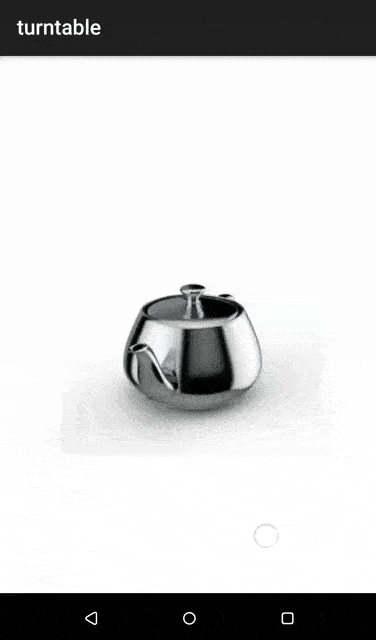# Create Turntable Animation with TitaniumGuest poster, Michael Gangolf, has been using Titanium since 2010, been a Titan since 2017 and Android lover since the beginning. You can follow him at @michaelgangolf or on Github at https://github.com/m1ga

Following the color animation tutorial, this post shows how to create turntable animation with Titanium. Turntable animation is a 360° animation in one axis:and is mostly used to show 3D objects from all sides. Since we cannot use a 3D engine, we will use a prerendered sprite sheet to create our animation. The sprite sheet contains all frames of the 360° animation and is stacked in a grid. The bigger you create the image, the higher the resolution will be; if you create more frames, you will have a smoother animation. In this example, we use a 8×8 grid with a 250x250px frame. So, the full image will be 2000x2000px.The Alloy setup is rather easy – a View with the whole image and one View around it that displays only one frame:

index.xml

``````<Alloy>
<Window>
<View id="frameView">
<View id="img"/>
</View>
</Window>
</Alloy>``````

index.tss

``````"#frameView" : {
width: 250,
height: 250,
touchEnabled: false
}
"#img" : {
width: 2000,
height: 2000,
backgroundImage: "/images/turntable.jpg",
touchEnabled: false,
top:0,
left:0
}``````

The whole logic is inside the controller where we use touchStart/touchMove to cycle through the frames. To do this, we have disabled `touchEnabled` on the two views and add two event listeners to our index window:

``````function onStart(e) {
}

function onMove(e) {
}

\$.index.open();``````

Inside onStart, we store the first x coordinate where the user clicks on the screen and calculate the distance to it every time touchmove is called:

``````var distX = 0;
var sX = 0;
var frame = 0;

function onStart(e) {
// set start value
sX = e.x;
}

function onMove(e) {
// calculate distance between start and current x
distX = (sX - e.x);
}

\$.index.open();``````

If we move 5px, we will change a frame and set the start point the the new x coordinate. When we reach frame 64, we have to go to frame 0 again and visa versa so we have an endless loop.

``````function onMove(e) {
// calculate distance between start and current x
distX = (sX - e.x);

// 5px buffer until we change a frame
if (distX < -5) {
frame--;
sX = Math.floor(e.x);
} else if (distX > 5) {
frame++;
sX = Math.floor(e.x);
}

// check frame
if (frame >= 64) {
frame = 0;
} else if (frame < 0) {
frame = 63;
}
}``````

The last thing we need to do is calculate the top and left position of our frame. Frames 1 to 8 are at top=0 and left=0, 250, 500,…. Once we reach frame 9, we will start from left=0 again, but move top one row down (250px). In Javascript, we can do this simply with:

``````// frame to px
var x = Math.floor((frame * 250) % 2000);
var y = Math.floor((frame * 250) / 2000) * 250;

// change image
\$.img.left = -x;
\$.img.top = -y;``````

When you start your app and move your finger from one side to another, you will see a spinning object! The whole index.js looks like this:

``````var distX = 0;
var sX = 0;
var frame = 0;

function onStart(e) {
// set start value
sX = e.x;
}

function onMove(e) {
// calculate distance between start and current x
distX = (sX - e.x);

// 5px buffer until we change a frame
if (distX < -5) {
frame--;
sX = Math.floor(e.x);
} else if (distX > 5) {
frame++;
sX = Math.floor(e.x);
}

// check frame
if (frame >= 64) {
frame = 0;
} else if (frame < 0) {
frame = 63;
}

// frame to px
var x = Math.floor((frame * 250) % 2000);
var y = Math.floor((frame * 250) / 2000) * 250;

// change image
\$.img.left = -x;
\$.img.top = -y;
}

\$.index.open();``````

and you can find the full project at: https://github.com/m1ga/appcelerator_turntable_animation1.said.C

Hi,

I don’t understand why you don’t use several images and change only the image path ? what is the advantage of this solution ?

•Michael

@said.C The advantage is that you have the whole image loaded and it won’t flicker. It give a much smoother experience and is very often used in game engines.

• I never knew this fact. I always think that user experience is the key in the whole app. and I will be in the front of the row when it comes down to the learning of new ways for the improvement of the user experience.

2.Zachary Litten

Gives me an idea of a way to implement a parallax effect on a background. Thanks for sharing!

•Michael

You’re welcome. Did a parallax effect, too: ScrollableView with content + scroll-event + multiple background layers that are moved with different parameters. Have fun playing around with it, can create some nice effects

3.Guile

For every touch move (or scroll) I would suggest to use a throttled function as event handler.
http://underscorejs.org/#throttle

With a delay between 40ms (25fps) and 20ms (50fps), it will improve code without slowing “animations”